by Andrew Miller
I am dedicated to helping my students be successful. Therefore, every student will receive the custom-tailored support n...

# How to Find the Area of a Shape

The term “area” in mathematics refers to the amount of space that a two-dimensional shape occupies. Area can be represented by centimeters, meters, feet, and other units of measurement. Because not all geometric 2D shapes have the same number of sides, a different formula is required for calculating the area for each shape. This page will focus on finding the area of seven of the most common 2D shapes. These include rectangle, square, circle, triangle, trapezoid, ellipse, and parallelogram.

Because these formulas involve 2D shapes, all area calculations have a “2” exponent (also known as a superscript or power) to indicate that the shape has two sides.

## How to Find the Area of a Rectangle

The formula for finding the area of a rectangle is A = w x l, where “w” represents the width and “l” represents the length.

Example:

A = 32 x 52

A = 1664 ft2

## How to Find the Area of a Square

Use the A = a2 formula to find the area of a square. The “a” represents one side of the square. Since a square has four equal sides, having the measurement of one side gives you the measurement for the others.

Example: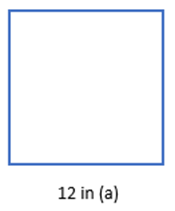A = a2

A = 122

A = 144 in2

## How to Find the Area of a Circle

To find the area of a circle, use the following formula: A = πr2. The “r” in this formula represents the radius of the circle.

Example: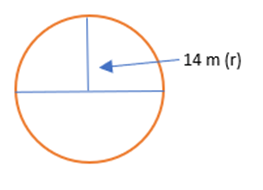A = π 2

A = 14π 2

A = π 196

A ≈ 615.75 m2

## How to Find the Area of a Triangle

The standard formula for finding the area of a triangle is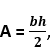where “b” represents the base and “h” represents the height.

Example: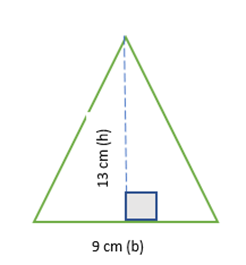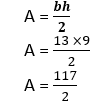A = 58.5 cm2

## How to Find the Area of a Trapezoid

Use the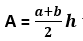formula to find the area of a trapezoid. In this formula, “a” represents the shorter base (top), “b” represents the longer base (bottom), and “h” represents the height.

Example: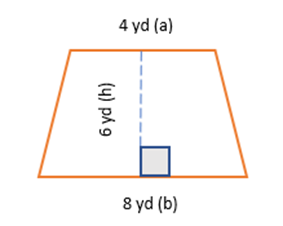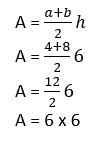A = 36 yd2

## How to Find the Area of an Ellipse

An ellipse is similar to a circle in its appearance, except that it is precisely defined. It is, essentially, a circle, stretched horizontally, with two symmetrical axes. An oval is not precisely defined. To calculate the area of an ellipse, use the A = π a x b formula. The “a” represents the horizontal axis while the “b” represents the vertical axis.

Example: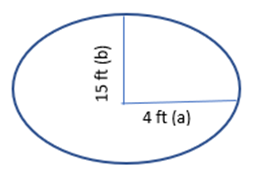A = π a x b

A = π x 4 x 15

A ≈ 12.566 x 15

A ≈ 188.5 ft2

## How to Find the Area of a Parallelogram

The formula for calculating the area of a parallelogram is A = b x h. The “b” represents the base, and the “b” represents the height.

Example: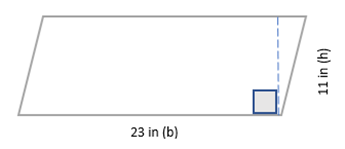A = b x h

A = 23 x 11

A = 253 in2

Area Formulas of Common Geometric Shapes

The chart below provides the area formula of the aforementioned 2D shapes, in addition to some other common ones.

### Top Posts

Education: College Majors with the Highest Salaries by Heytutor Blog
Education: States with the Largest Teacher Pay Gap by Heytutor Blog